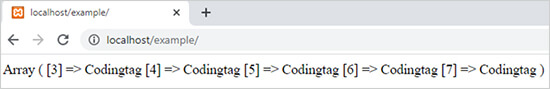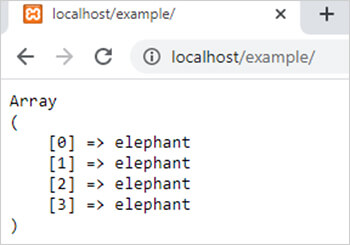array_fill() function in php || PHP Functions

array_fill() function in php

0 1151

array_fill() function of PHP is used to fill an array with values means it generates an array by values provide by user. It is an inbuilt function of PHP.

Syntax:

array_fill (\$starting_index, \$number_of_elements, \$array_value);

Here

1. \$starting_index is the initial index of array.
2. \$number_of_elements represents the number of elements in the array.
3. \$array_value is the value of every element of array.

Example 1:

<?php
\$index=3;
\$no_of_elemnts=5;
\$arr_value="Codingtag";
\$arr1=array_fill(\$index,\$no_of_elemnts,\$arr_value);
print_r(\$arr1);
?>

Output:Example 2:

<?php
\$index=0;
\$no_of_elemnts=4;
\$arr_value="elephant";
\$arr1=array_fill(\$index,\$no_of_elemnts,\$arr_value);
echo "<pre>";
print_r(\$arr1);
?>

Output:Share: